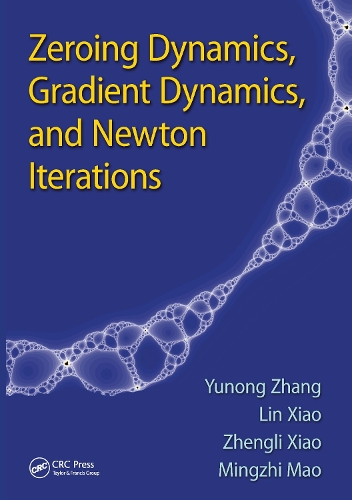•# Zeroing Dynamics, Gradient Dynamics, and Newton Iterations (Paperback)

(author), (author), (author), (author)
£60.00
Paperback 310 Pages / Published: 01/01/2021
• We can order this

Usually dispatched within 1 week

Neural networks and neural dynamics are powerful approaches for the online solution of mathematical problems arising in many areas of science, engineering, and business. Compared with conventional gradient neural networks that only deal with static problems of constant coefficient matrices and vectors, the authors' new method called zeroing dynamics solves time-varying problems.

Zeroing Dynamics, Gradient Dynamics, and Newton Iterations is the first book that shows how to accurately and efficiently solve time-varying problems in real-time or online using continuous- or discrete-time zeroing dynamics. The book brings together research in the developing fields of neural networks, neural dynamics, computer mathematics, numerical algorithms, time-varying computation and optimization, simulation and modeling, analog and digital hardware, and fractals.

The authors provide a comprehensive treatment of the theory of both static and dynamic neural networks. Readers will discover how novel theoretical results have been successfully applied to many practical problems. The authors develop, analyze, model, simulate, and compare zeroing dynamics models for the online solution of numerous time-varying problems, such as root finding, nonlinear equation solving, matrix inversion, matrix square root finding, quadratic optimization, and inequality solving.

Publisher: Taylor & Francis Ltd
ISBN: 9781138894082
Number of pages: 310
Dimensions: 254 x 178 mm